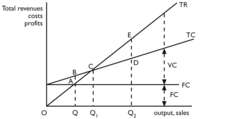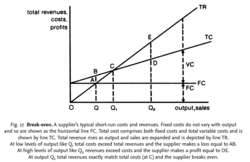# break-even

(redirected from break-evens)
Also found in: Dictionary, Encyclopedia.

## Break Even

1. To make the sales or revenues necessary to cover costs and prevent a firm from operating at a loss. The breakeven may be relatively stable or it may fluctuate, depending on the company or industry. Companies with high breakevens tend to have large fluctuations in earnings from year to year.

2. To sell a security at a price that causes the seller to neither make a profit nor lose money on the sale.Fig. 11 Break-even. A supplier's typical short-run costs and revenues. Fixed costs do not vary with output and so are shown as the horizontal line FC. Total cost comprises both fixed costs and total variable costs and is shown by line TC. Total revenue rises as output and sales are expanded and is depicted by line TR. At low levels of output such as Q total costs exceed total revenues and the supplier makes a loss equal to AB. At high levels of output such as Q2 revenues exceed costs and the supplier makes a profit equal to DE. At output Q1 total revenues exactly match total costs (at C) and the supplier breaks even.

## break-even

the short-run rate of output and sales at which a supplier generates just enough revenue to cover his fixed and variable costs, earning neither a PROFIT nor a LOSS. If the selling price of a product exceeds its unit VARIABLE COST then each unit of product sold will earn a CONTRIBUTION towards FIXED COSTS and profits. Once sufficient units are being sold so that their total contributions cover the supplier's fixed costs then the company breaks even. If less than the break-even sales volume is achieved then total contributions will not meet fixed costs and the supplier will make a loss. If the sales volume achieved exceeds the break-even volume, total contributions will cover the fixed costs and leave a surplus which constitutes profit. See Fig. 11.

Differences in cost structures can have a significant effect upon companies' break-even points. For example, a company with low levels of automation and so little capital equipment (for example Rolls Royce cars) would have low fixed depreciation costs but high direct labour costs. With high unit direct costs relative to selling prices such a firm would have a low unit contribution but low fixed costs, so would break even at a low sales volume, though profits would climb only slowly beyond the break-even point because of low unit contribution. By contrast, a highly automated plant would have high fixed depreciation costs, but with low unit direct labour costs, would have a higher unit contribution. Firms with such plants (for example Ford) would not break even until a much higher sales volume was achieved, but thereafter profits would increase rapidly with larger unit contributions. See MARGINAL COSTING, PROFIT-VOLUME CHART.Fig. 17 Break-even. A supplier's typical short-run costs and revenues. Fixed costs do not vary with output and so are shown as the horizontal line FC. Total cost comprises both fixed costs and total variable costs and is shown by line TC. Total revenue rises as output and sales are expanded and is depicted by line TR. At low levels of output like Q, total costs exceed total revenues and the supplier makes a loss equal to AB. At high levels of output like Q2 revenues exceed costs and the supplier makes a profit equal to DE. At output Q1 total revenues exactly match total costs (at C) and the supplier breaks even.

## break-even

the short-run rate of output and sales at which a supplier generates just enough revenue to cover his fixed and variable costs, earning neither a PROFIT nor a LOSS. If the selling price of a product exceeds its unit VARIABLE COST, then each unit of product sold will earn a CONTRIBUTION towards FIXED COSTS and profits. Once sufficient units are being sold so that their total contributions cover the supplier's fixed costs, then the company breaks even. If less than the break-even sales volume is achieved, then total contributions will not meet fixed costs and the supplier will make a loss. If the sales volume achieved exceeds the breakeven volume, total contributions will cover the fixed costs and leave a surplus that constitutes profit.
References in periodicals archive ?
According to Deutsche Bank's calculations, break-even oil prices of both Saudi Arabia and the UAE have fallen for the first time in the past few years.
"The average break-even price in the GCC countries will edge down to about \$80 barrels this year, the first drop since 2003," Deutsche Bank's Robert Burgess wrote in a note to clients.
The bank said Saudi Arabia's break-even price has fallen from \$82.2 to \$78.4, while the UAE's is more pronounced from \$99.1 to \$90, as fiscal pressures ease in both countries.

Site: Follow: Share:
Open / Close